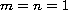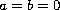Electron. J. Diff. Eqns., Vol. 2009(2009), No. 65, pp. 1-16.

### Oblique derivative problems for generalized Rassias equations of mixed type with several characteristic boundaries Guo Chun Wen

Abstract:
This article concerns the oblique derivative problems for second-order quasilinear degenerate equations of mixed type with several characteristic boundaries, which include the Tricomi problem as a special case. First we formulate the problem and obtain estimates of its solutions, then we show the existence of solutions by the successive iterations and the Leray-Schauder theorem. We use a complex analytic method: elliptic complex functions are used in the elliptic domain, and hyperbolic complex functions in the hyperbolic domain, such that second-order equations of mixed type with degenerate curve are reduced to the first order mixed complex equations with singular coefficients. An application of the complex analytic method, solves (1.1) below with,, which was posed as an open problem by Rassias.

Submitted December 16, 2008. Published May 14, 2009.
Math Subject Classifications: 35M05, 35J70, 35L80.
Key Words: Oblique derivative problems; generalized Rassias equations; several characteristic boundaries.

Show me the PDF file (286 KB), TEX file, and other files for this article.Guo Chun Wen LMAM, School of Mathematical Sciences Peking University, Beijing 100871, China email: Wengc@math.pku.edu.cn